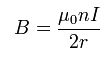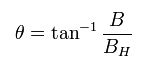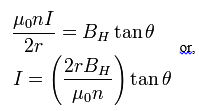0
Total:   \$0.00 details
There are no products in your shopping cart!
We hope it's not for long.

## Voyager Rare Books Maps & Prints

A tangent galvanometer is an early measuring instrument used for the measurement of electric current. It works by using a compass needle to compare a magnetic field generated by the unknown current to the magnetic field of the Earth. It gets its name from its operating principle, the tangent law of magnetism, which states that the tangent of the angle a compass needle makes is proportional to the ratio of the strengths of the two perpendicular magnetic fields. It was first described by Claude Pouillet in 1837.

A tangent galvanometer consists of a coil of insulated copper wire wound on a circular non-magnetic frame. The frame is mounted vertically on a horizontal base provided with leveling screws. The coil can be rotated on a vertical axis passing through its centre. A compass box is mounted horizontally at the centre of a circular scale. It consists of a tiny, powerful magnetic needle pivoted at the centre of the coil. The magnetic needle is free to rotate in the horizontal plane. A long thin pointer is attached to the needle at its centre and at right angle to it.

In operation, the instrument is first rotated until the magnetic field of the Earth, indicated by the compass needle, is parallel with the plane of the coil. Then the unknown current is applied to the coil. This creates a second magnetic field on the axis of the coil, perpendicular to the Earth’s magnetic field. The compass needle responds to the vector sum of the two fields, and deflects to an angle equal to the tangent of the ratio of the two fields. From the angle read from the compass’s scale, the current could be found from a table. The current supply wires have to be wound in a small helix, like a pig’s tail, otherwise the field from the wire will affect the needle and an incorrect reading will be obtained.

### Theory

The galvanometer is oriented so that the plane of the coil is vertical and aligned along parallel to the horizontal component of the Earth’s magnetic field (i.e. parallel to the local “magnetic meridian”). When an electrical current flows through the galvanometer coil, a second magnetic field B is created. At the center of the coil, where the compass needle is located, the coil’s field is perpendicular to the plane of the coil. The magnitude of the coil’s field is:where I is the current in amperes, n is the number of turns of the coil and r is the radius of the coil. These two perpendicular magnetic fields add vectorially, and the compass needle points along the direction of their resultant BH+B. The current in the coil causes the needle to rotate by an angle θ:From tangent law, B = BH tan θ, i.e.or I = K tan θ, where K is called the Reduction Factor of the tangent galvanometer.